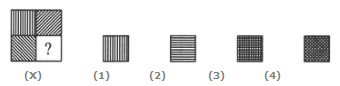Direction In each of the following questions, select a figure from amongst the four alternatives, which when placed in the blank space of figure (X) would complete the pattern..
1.

Identify the figure that completes the pattern.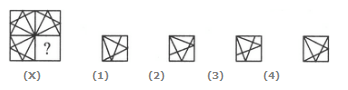A. 1 B. 2 C. 3 D. 4
Explanation:

2.

Identify the figure that completes the pattern.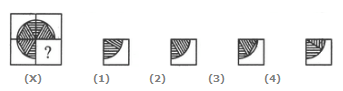A. 1 B. 2 C. 3 D. 4
Explanation:

3.

Identify the figure that completes the pattern.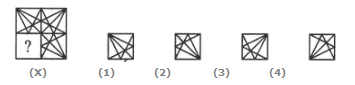A. 1 B. 2 C. 3 D. 4
Explanation:

4.

Identify the figure that completes the pattern.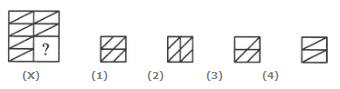A. 1 B. 2 C. 3 D. 4